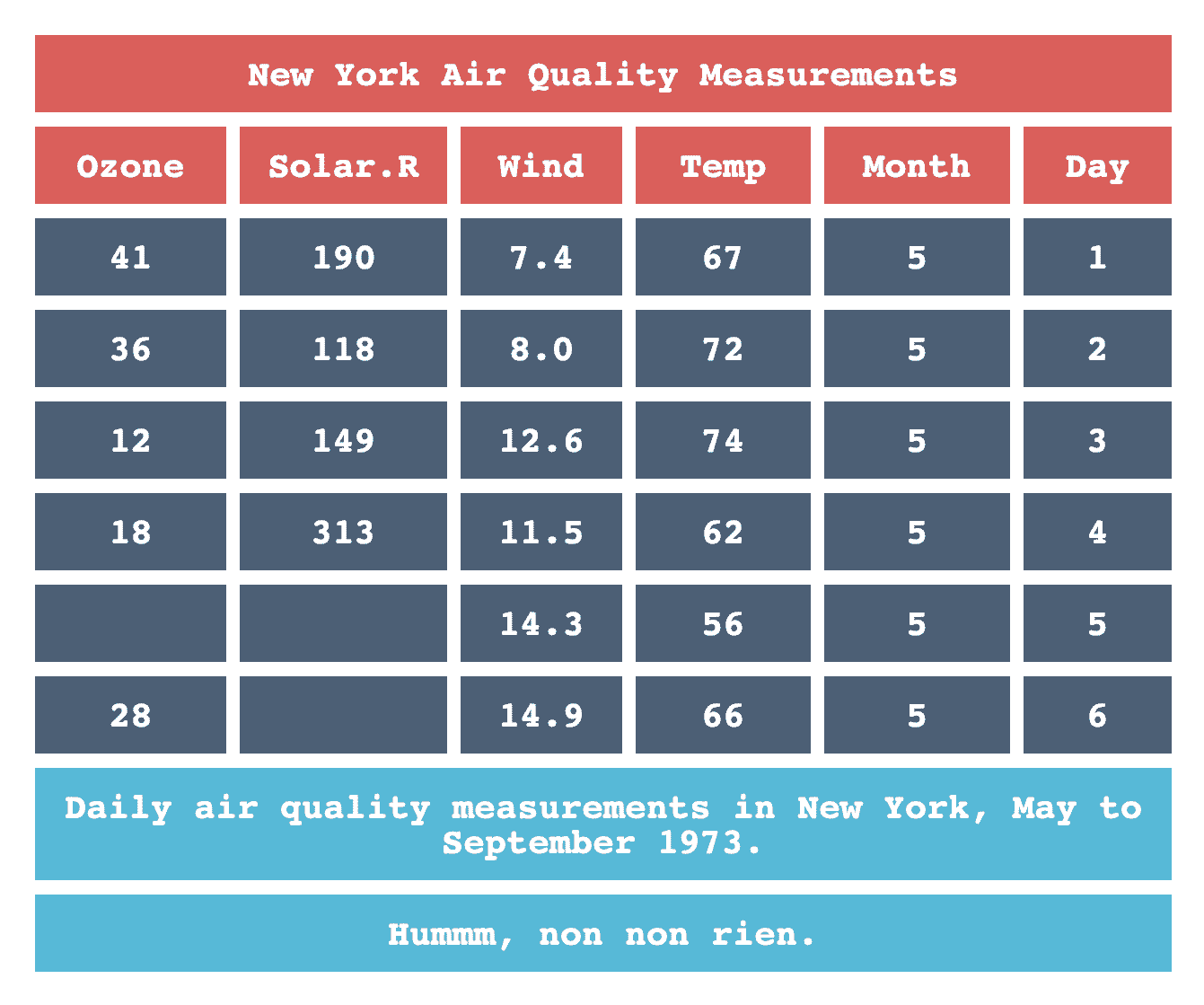# Creating a theme function

``````library(flextable)
library(magrittr)``````

In this example, a theme function is created and used to format different `data.frame`.

A theme function is a R function where input is a flextable and output is a flextable. You are free to do any formatting inside this function.

``````theme_design <- function(x) {
x <- border_remove(x)
std_border <- fp_border_default(width = 4, color = "white")
x <- fontsize(x, size = 10, part = "all")
x <- font(x, fontname = "Courier", part = "all")
x <- align(x, align = "center", part = "all")
x <- bold(x, bold = TRUE, part = "all")
x <- bg(x, bg = "#475f77", part = "body")
x <- bg(x, bg = "#eb5555", part = "header")
x <- bg(x, bg = "#1bbbda", part = "footer")
x <- color(x, color = "white", part = "all")
x <- border_outer(x, part="all", border = std_border )
x <- border_inner_h(x, border = std_border, part="all")
x <- border_inner_v(x, border = std_border, part="all")
x <- set_table_properties(x, layout = "fixed")
x
}``````
``````flextable(head(cars)) %>%
theme_design()``````
 speed dist 4 2 4 10 7 4 7 22 8 16 9 10
``````ft <- flextable(head(airquality)) %>%